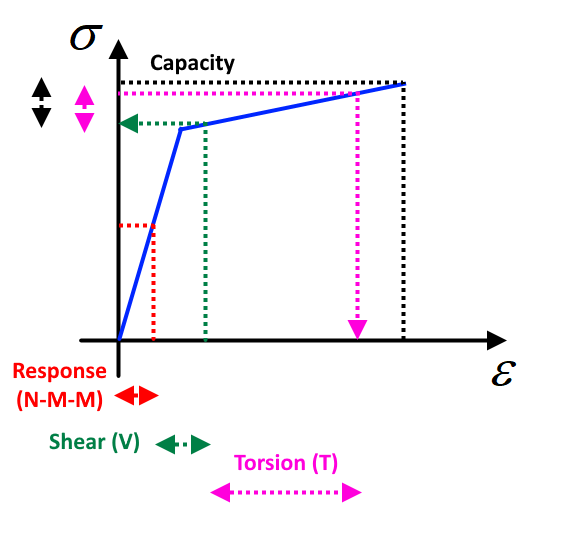### Choose language# How is the interaction check calculated?

Interaction check verifies a cross-section capacity to carry additional forces due to shear and torsion. Both concrete and reinforcement parts must safely transfer compression and tension caused by the load effects.
$$The cross-section can be subjected to the normal force, bending moments and shear forces simultaneously (N-M-M + V). In complex structures such as bridges, even another component, the torsion moment (T), can act in the cross-section. That is why we need to take all these components (N-M-M + V + T) into account and check the cross-section capacity.Interaction check is about checking the concrete in compression, verifying a reinforcement capacity to carry additional forces due to shear and torsion in longitudinal bars, and checking the combination of shear and torsion in transverse bars (stirrups). Let's explain in detail how the interaction check of longitudinal reinforcement is done in IDEA StatiCa RCS. The principle of interaction check is based on the response of the cross-section due to acting bending moments and normal force (N-M-M). Once the response is calculated, you can add the increments of tensile forces due to shear and (or) torsion to longitudinal reinforcement and plot them into stress-strain diagrams of reinforcing steel. If you know the strain due to shear and (or) torsion, you can easily find out whether there is a reserve in the reinforcing bar or not.$$